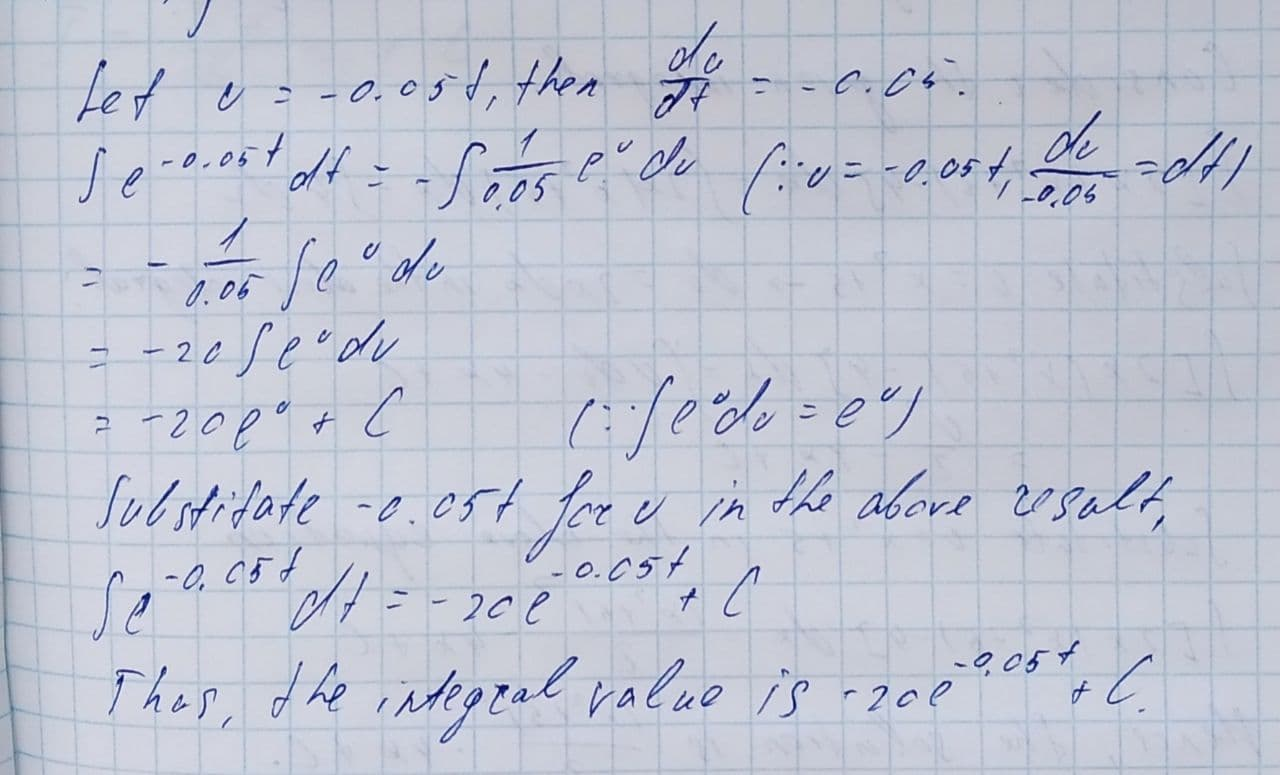Question# Find the indefinite integral. \int e^{-0.05t}dt

Applications of integrals
ANSWEREDFind the indefinite integral.
$$\displaystyle\int{e}^{{-{0.05}{t}}}{\left.{d}{t}\right.}$$